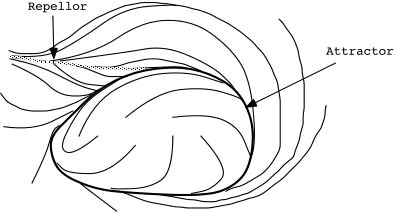Thoughts on the Edge of Chaos

M.M.Taylor and R.A. Pigeau

1. Overview

2. Basic Ideas: Information and structure, Attractors and Repellors

3. Basic Ideas: Catastrophe

4. Structure and Chaos

5. Six Kinds of Replication

6. Categories and Logic

7. Surprise and importing structure

8. Replication

### Six kinds of Replication

Imagine a one-dimensional state space at time t 0. Each state defines a trajectory over time, so that the states at t 0 will map into new states (in the same space) at t 1. Without mathematical rigour, we assert that there are 6 interestingly different kinds of map, of which only one will be interesting as a model of cognitive function. The six kinds of map are illustrated in Fig 6.The first kind of map (Fig.6 top-left) is random, in the sense that no matter how small delta t = t1- t0, knowledge of the state at t 0 gives no information about the state at t 1. We assert that such maps cannot occur in physical systems.

At the other end of the scale (Fig.6 bottom-right) lies a map we call "death", in which state transitions are limited to fixed or periodic attractors. All dissipative systems lead to such maps in the absence of information-carrying input from outside the system. This map is physically possible, but uninteresting.

Between the random map and the map of death lie four other maps: we call them "random chaos" (Fig.6 top-right), "ordinary chaos" (Fig.6 middle-left), "semi-chaos" (Fig.6 middle-right), and "logic (Fig.6 bottom-left). The distinctions among these different maps are described below. We assert that logic cannot occur in systems with a material substrate, and that semi-chaos is the only map of interest in cognitive systems.

Chaotic maps are distinguished by the fact that if the initial state is known exactly, future states are exactly predictable, but if there is any uncertainty about the initial state, the trajectories of the states within the region of uncertainty diverge exponentially. Very loosely, the rate of divergence is called the Lyapunov exponent of the chaotic system. The actual definition of the Lyapunov exponent refers to the limit of the trajectory after infinite time, but it is convenient for our purposes to use an analogous concept, defined near time t 0, which we will call the "local divergence."Fig.7 Illustrating the concept of an attractor and a repellor. Every point in this figue is in the basin of attraction of the single attractor.

If the trajectories converge, the Lyapunov exponent is negative, and the state is in the basin of attraction of some attractor. The local divergence may be positive or negative within a basin of attraction, but after sufficient time they become negative as the trajectories converge to the attractor (Fig.7). In a multidimensional state space, the trajectories may converge for states varying in one direction, but diverge for states varying in an orthogonal direction (Fig.8). Accordingly, each state in a multidimensional state space has as many Lyapunov exponents as there are dimensions.Fig 8. A system in which the dynamic has one positive and one negative Lyapunov exponent.

In a map of random chaos, the Lyapunov exponents are everywhere positive, and there are no attractors. Randomly chaotic maps are mathematically possible (they occur in the idealized "billiard-ball atom" model of statistical thermodynamics), but are not interesting in the present context, since they deny the possibility of any stable regions that could relate to categories of thought.

Ordinary chaos (Fig 6 middle-left) seems to be the most common kind of map in the natural world. The state space has regions of divergence and regions of convergence, which means that almost all states are in the basin of attraction of some attractor. Although over much of the state space the basins of different attractors are thoroughly mixed, so that slight uncertainties in the initial state include trajectories leading to different attractors, nevertheless some prediction is possible in regions where the local divergences and the Lyapunov exponents are negative.

Mathematically identical to ordinary chaos is what we call semi-chaos (Fig. 6 middle-right). The difference is in the way the basins of attraction are distributed. In a semi-chaotic map, relatively large regions of the state space fall within individual basins of attraction, and only in small regions are the local divergences positive. These boundary regions between basins of attraction may well have a fractal geometric character, but at any scale comparable to the uncertainty of measurement of the initial state, they occupy a relatively small region of the state space. In a semi-chaotic map, states may be segregated into categories with ill-defined boundaries. Within a category, all states have trajectories leading to the same attractor, and we will identify this attractor as an ideal member of the category.

The final map (Fig. 6 bottom-left) is the one we call "logic". A logical map is an extreme version of the semi-chaotic map, in which the boundaries between attractor basins are well-defined. No matter what the state, there is no uncertainty about which category it belongs to. We will add the proviso that within the category there is no discrimination among states, so that although all the trajectories within a category lead through the same future sequence of categories, there no information is available about which states are occupied within the categories. To give a concrete example, a logic gate in a computer is defined to be in a 1 state if the voltage is between x and x plus delta x and in a 0 state if the voltage is between y and y plus delta y, two non-overlapping ranges of voltage. The electronic circuitry prohibits other voltages (except as vanishingly brief transients during the switch between states). No part of the logic associated with the gate is influenced by the actual voltage, only by whether it is in a 1 or a 0 state.

Top of Page